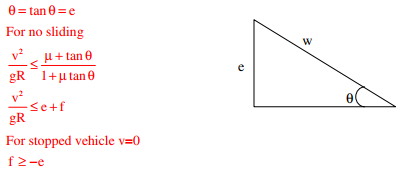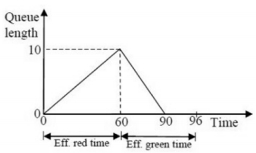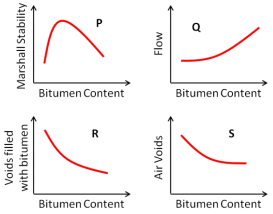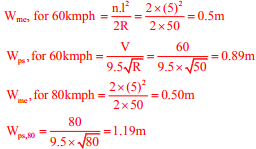# Transportation Engineering - Online Test

Q1. A runway is being constructed in a new airport as per the International Civil Aviation Organization (ICAO) recommendations. The elevation and the airport reference temperature of this airport are 535 m above the mean sea level and 22.65°C, respectively. Consider the effective gradient of runway as 1%. The length of runway required for a design-aircraft under the standard conditions is 2000 m. Within the framework of applying sequential corrections as per the ICAO recommendations, the length of runway corrected for the temperature is
Explaination / Solution:

Q2. A super-elevation e is provided on a circular horizontal curve such that a vehicle can be stopped on the curve without sliding. Assuming a design speed v and maximum coefficient of side friction fmax, which one of the following criteria should be satisfied?
Explaination / Solution:Q3. The accuracy of an Electronic Distance Measuring Instrument (EDMI) is specified as ± (a mm + b ppm). Which one of the following statements is correct?
Explaination / Solution:

Accuracy of EDMI is generally stated in terms of constant instrument error and measuring error proportional to distance being measured. ± (a mm + b ppm) The first part in this expression indicates a constant instrument error that is independent of length of line measured. Second component is distance related error.

Q4. The queue length (in number of vehicles) versus time (in seconds) plot for an approach to a signalized intersection with the cycle length of 96 seconds is shown in the figure (not drawn to scale).At time t = 0, the light has just turned red. The effective green time is 36 seconds, during which vehicles discharge at the saturation flow rate, s (in vph). Vehicles arrive at a uniform rate v (in vph), throughout the cycle. Which one of the following statements is TRUE?

Explaination / Solution:
No Explaination.

Q5. The radius of a horizontal circular curve on a highway is 120 m. The design speed is 60 km/hour, and the design coefficient of lateral friction between the tyre and the road surface is 0.15. The estimated value of superelevation required (if full lateral friction is assumed to develop), and the value of coefficient of friction needed (if no superelevation is provided) will, respectively, be
Explaination / Solution:Q6. During a forensic investigation of pavement failure, an engineer reconstructed the graphs P, Q, R and S, using partial and damaged old reports.Theoretically plausible correct graphs according to the 'Marshall mixture design output' are
Explaination / Solution:
No Explaination.

Q7. A two lane, one-way road with radius of 50 m is predominantly carrying lorries with wheelbase of 5 m. The speed of lorries is restricted to be between 60 kmph and 80 kmph. The mechanical widening and psychological widening required at 60 kmph are designated as wme,80 and wps,80, respectively. The mechanical widening and psychological widening required at 80 kmph are designated as and wme,80 and wps,80, respectively. The correct values of wme,60wps,60 respectively are
Explaination / Solution:Q8.  The following statements are made related to the lengths of turning lanes at signalised intersections
(i) 1.5 times the average number of vehicles (by vehicle type) that would store in turning lane per cycle during the peak hour.
(ii) 2 times the average number of vehicles (by vehicle type) that would store in turning lane per cycle during the peak hour.
(iii) Average number of vehicles (by vehicle type) that would store in the adjacent through lane per cycle during the peak hour.
(iv) Average number of vehicles (by vehicle type) that would store in all lanes per cycle during the peak hour.
As per the IRC recommendations, the correct choice for design length of storage lanes is
Explaination / Solution:
No Explaination.

Q9. A road is being designed for a speed of 110 km/hr on a horizontal curve with a super elevation of 8%. If the coefficient of side friction is 0.10, the minimum radius of the curve (in m) required for safe vehicular movement is
Explaination / Solution:

= (110×110)/(127(0.08+0.10)) = 529.30m

Q10. On a section of a highway the speed-density relationship is linear and is given by v = [80-(2/3)k]; where v is in km/h and k is in veh/km. The capacity (in veh/h) of this section of the highway would be
Explaination / Solution: